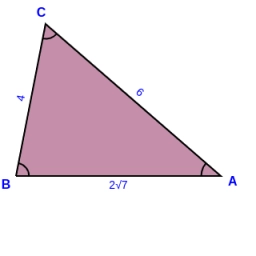# Circumscribed 81759

In triangle ABC, we know a = 4 cm, b = 6 cm, γ = 60°. Calculate the area and radius of the inscribed and circumscribed circle.

S =  10.3923 cm2
r =  1.3592 cm
R =  3.0551 cm

### Step-by-step explanation:

Try calculation via our triangle calculator.Did you find an error or inaccuracy? Feel free to write us. Thank you!

Tips for related online calculators
The Pythagorean theorem is the base for the right triangle calculator.
Do you want to convert time units like minutes to seconds?
Cosine rule uses trigonometric SAS triangle calculator.